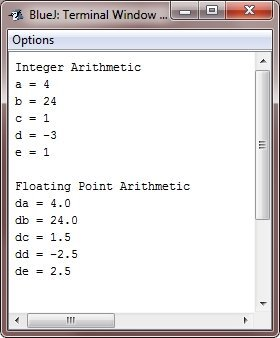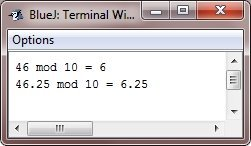# Java Arithmetic and Modulus Operator

## Arithmetic Operators

Arithmetic operators are used in the mathematical expressions in same way that they are used in algebra.

### All Arithmetic Operators List

The following table lists all the arithmetic operators :

Operator Name
- Subtraction (unary minus)
* Multiplication
/ Division
% Modulus
++ Increment
-= Subtraction assignment
*= Multiplication assignment
/= Division assignment
%= Modulus assignment
-- Decrement

The operands in arithmetic operators must be of a numeric type. You cannot use them on boolean types, but you can use them on the char types, since the char type in Java is, essentially a subset of int.

### Basic Arithmetic Operators

The basic arithmetic operations are:

• subtraction operator
• multiplication operator
• division operator

all acts as you would expect for all the numeric types.

The unary minus operator negates its single operand. The unary plus operator returns the value of its operand. Always remember that when the division operator is applied to an integer type, there will be no fractional component connected to the result.

### Example

The following example program illustrates the arithmetic operators. It also illustrates the difference between floating-point division and integer division.

```/* Java Program Example - Java Arithmetic Operators
*  This program demonstrates the basic arithmetic operators. */

public class JavaProgram
{
public static void main(String args[])
{

/* arithmetic using integers */
System.out.println("Integer Arithmetic");
int a = 2 + 2;
int b = a * 6;
int c = b / 16;
int d = c - a;
int e = -d;
System.out.println("a = " + a);
System.out.println("b = " + b);
System.out.println("c = " + c);
System.out.println("d = " + d);
System.out.println("e = " + c);

/* arithmetic using doubles */
System.out.println("\nFloating Point Arithmetic");
double da = 2 + 2;
double db = da * 6;
double dc = db / 16;
double dd = dc - da;
double de = -dd;
System.out.println("da = " + da);
System.out.println("db = " + db);
System.out.println("dc = " + dc);
System.out.println("dd = " + dd);
System.out.println("de = " + de);

}
}```

When the above Java program is compile and executed, it will produce the following output:## The Modulus Operator

The modulus operator (%) is a useful operator in Java, it returns the remainder of a division operation. It can be applied to the floating-point types and integer types both.

### Example

The following example program illustrates the modulus operator (%) :

```/* Java Program Example - Java Arithmetic Operators
*  This program demonstrates the modulus operator (%) */

public class JavaProgram
{
public static void main(String args[])
{

int x = 46;
double y = 46.25;

System.out.println("46 mod 10 = " + x%10);
System.out.println("46.25 mod 10 = " +y%10);

}
}```

When the above Java program is compile and executed, it will produce the following output:### Arithmetic Compound Assignment Operators

Java allows special operators that can be used to combine an arithmetic operations with an assignment. As you probably know, statements like this are quite common in programming:

`a = a + 4;`

In Java, you can rewrite this statement as:

`a += 4;`

There are compound assignment operators for all of the arithmetic, binary operators. Thus, any statement of the form

`var = var op expression;`

can be rewritten as:

`var op= expression;`

### Example

Following is a simple Java program that shows several op= assignment in action:

```/* Java Program Example - Java Arithmetic Operators
*  This program demonstrates the several assignment operators. */

public class JavaProgram
{
public static void main(String args[])
{

int a = 1;
int b = 2;
int c = 3;

a += 10;
b *= 4;
c += a*b;
c %= 6;
System.out.println("a = " + a);
System.out.println("b = " + b);
System.out.println("c = " + c);

}
}```

When the above Java program is compile and executed, it will produce the following output:## Example

Here is some examples based on arithmetic operators and modulus operator, that you can go for.

Tools
Calculator